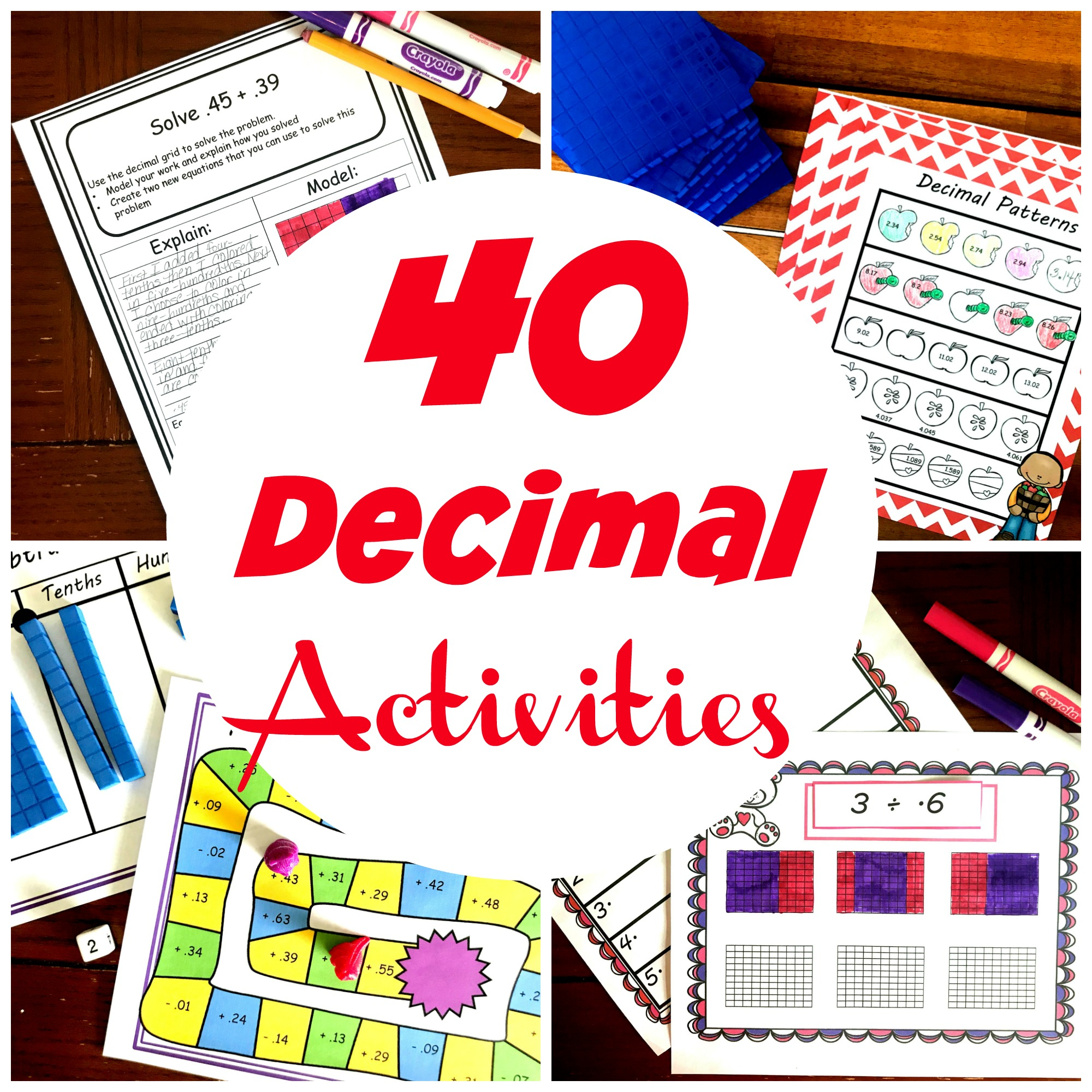# 40 Decimal Activities | Free | Hands On | Fun

## Fun Decimal Activities

Learning decimals, and converting decimals to fractions can be a scary concept for students. I mean, learning 3/8 as a decimal or 1/6 as a decimal means you are in real-deal math now. None of this easy addition and subtraction stuff.

Converting fractions to decimals and decimals to fractions are necessary life skills though. And must be learned. But, no worries. This list of fun decimal activities starts with easy decimal activities and then moves on to multiplication and division of decimals.

So, I’ve gathered the best hands on and fun decimal activities for elementary students.

Your children will love these engaging decimal activities. They are designed to strengthen students understanding of decimals as they add, subtract, multiply, and divide them.

As always, don’t forget to check out my assortment of activities on TeachersPayTeachers.

## Fun Ways to Teach Decimals

It may be tempting to just jump in and starting adding and subtracting decimals, but I highly suggest a little time of exploration first. These decimal activities are a great place to start.

First, let children play with base ten blocks, draw, color, and create number lines using decimals. These hands on decimal activities will give a multisensory learning experience.

All of these easy decimal activities will lay a strong foundation for the harder work to come.

You will be glad you took the time to do it!

## Fun Activities for Adding Decimals

Now it is time for a little adding. Once again it is essential that children get to see how adding decimals works before they work out problems. I love using base ten blocks, but if that doesn’t work for a child, number lines or decimal grids may provide the needed support!

## Subtracting Decimals

Next, we move into subtracting decimals. Subtraction tends to be so much harder for our little ones, and they will need lots of hands-on experience.

## Multiplying Decimals

Ahhhh, the challenges of multiplying decimals. And the all-time favorite, where does that decimal go?

I know our big kiddos like to just jump in and solve, but getting them in the habit of estimating first is a huge step. If they estimate correctly, they will know where that tricky little decimal should be.

Check out these multiplying decimal activities.

## Dividing Decimals Activities

Finally, we get to dividing decimals.

I hope you and your students enjoy these. You’ve got this!